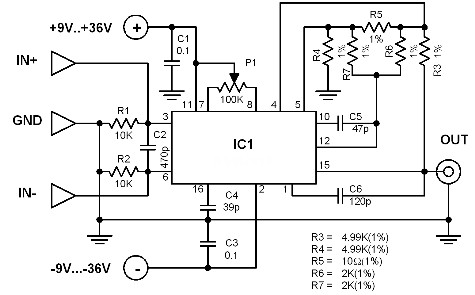# Low Noise Preamp Circuit Diagram

Low Noise Preamp Circuit Diagram This is a single IC low noise preamp made possible by using the SSM2016 from PMI. It has a symmetrical input and extremely low noise characteristics. It is highly capable of amplifying signal sources with low internal resistance (less than 1 kiloohms) like moving coil microphones and dynamic microphones with impedances ranging from 10 ohms to 600 ohms.Low Noise Preamp Circuit Diagram

### Low Noise Preamp Circuit Diagram

This is a single IC low noise preamp made possible by using the SSM2016 from PMI. It has a symmetrical input and extremely low noise characteristics.

It is highly capable of amplifying signal sources with low internal resistance (less than 1 kiloohms) like moving coil microphones and dynamic microphones with impedances ranging from 10 ohms to 600 ohms.

The amplification is adjustable by changing the resistance of R5 with a value between 3.5 and 1000 ohms. The amplification A can be found with the ff: formula:

A = (R3+R4)/R5+(R3+R4)/(R6+R79)

It can be simplified to A= 10K/R+3.5 using the given component values. If for example R5 = 10 ohms, then the amplification is 1000.

The offset voltage at the inputs can be set to zero through potentiomer P1. It is highly recommended to use metal film resistors in constructing the circuit.